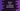# How to calculate permutation in Python## Finding permutation in python :

Python provides one utility method to find out the permutation of a iterable. This method is defined in itertool package. We can import this package and find out the permutation of any iterable like a dictionary, set or list elements.

### What is permutation :

Permutation is the arrangement of the elements. It can be the whole set of elements or a less number of elements. If we have n number of elements and if we are doing permutation of r elements, the formula to find out the total permutation is npr. It is equal to n!/(n-r)!.

So, for n elements, if we are doing permutation taking n elements on each, we can have n!/(n - n)! or n! permutations.

For example, for the string 123, we can have 3! = 6 permutations : 123, 132, 213, 231, 312, 321.

### Definition of itertool permutation :

The itertool permutation is defined as below :

``itertool.permutation(iterable[,r])``

Here, r is the length of each permutation. This is optional. If we don’t provide, or if we provide None, it will use the length of the iterable as the length.

### Example of permutation of string characters :

Let’s take a look at the below example :

``````import itertools as it

print(list(it.permutations('abc')))
print(list(it.permutations('abc',2)))``````

Here, we are importing the itertools package and using it to find out the permutations of the characters of ‘abc’.

The first print statement finds out the permutations of ‘abc’ by taking total characters as 3 for each values. The second print statement finds out the permutations of ‘abc’ by taking total characters as 2.

It will print the below output :

``````[('a', 'b', 'c'), ('a', 'c', 'b'), ('b', 'a', 'c'), ('b', 'c', 'a'), ('c', 'a', 'b'), ('c', 'b', 'a')]
[('a', 'b'), ('a', 'c'), ('b', 'a'), ('b', 'c'), ('c', 'a'), ('c', 'b')]``````

### Permutation of a list :

In the below example, we are using one list and finding out the permutations of the values :

``````import itertools as it

given_list = [1,2,3]

permutation = it.permutations(given_list)

for item in list(permutation):
print(item)``````

Output :

``````(1, 2, 3)
(1, 3, 2)
(2, 1, 3)
(2, 3, 1)
(3, 1, 2)
(3, 2, 1)``````

### Permutation of a list with duplicate values :

What would be the output if we have duplicate values in a list ? Let’s consider the below example :

``````import itertools as it

given_list = [1,1,3]

permutation = it.permutations(given_list)

for item in list(permutation):
print(item)``````

It will print :

``````(1, 1, 3)
(1, 3, 1)
(1, 1, 3)
(1, 3, 1)
(3, 1, 1)
(3, 1, 1)``````

Here, (1, 1, 3), (1, 3, 1) and (3, 1, 1) are repeated twice each. This is because the elements are treated as equal based on their position, not on their values. So, even if we have two elements with equal values at two different positions, they are treated as different values.

### Permuation of a tuple :

Now, let’s find out the permutation of a tuple elements :

``````import itertools as it

given_tuple = ('dog', 'cat', 'goat', 'lion')

permutation = it.permutations(given_tuple, 2)

for item in list(permutation):
print(item)``````

Here, we have created one tuple of four strings. But the permutations have two members each. It will give the below output :

``````('dog', 'cat')
('dog', 'goat')
('dog', 'lion')
('cat', 'dog')
('cat', 'goat')
('cat', 'lion')
('goat', 'dog')
('goat', 'cat')
('goat', 'lion')
('lion', 'dog')
('lion', 'cat')
('lion', 'goat')``````

### Permutations of a dictionary :

``````import itertools as it

given_dictionary = {
1: 'one',
2: 'two',
3: 'three'
}

permutation = it.permutations(given_dictionary)

for item in list(permutation):
print(item)``````

It will print :

``````(1, 2, 3)
(1, 3, 2)
(2, 1, 3)
(2, 3, 1)
(3, 1, 2)
(3, 2, 1)``````

As you can see here, the permutation is created using only the keys. We can use these keys to get the values of the dictionary.